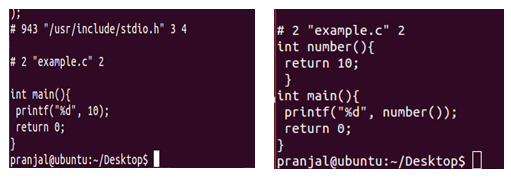# Macros vs Functions

• Difficulty Level : Easy
• Last Updated : 22 Jun, 2022

A macro is a name given to a block of C statements as a pre-processor directive. Being a pre-processor, the block of code is communicated to the compiler before entering into the actual coding (main () function). A macro is defined with the pre-processor directive. Macros are pre-processed which means that all the macros would be processed before your program compiles. However, functions are not preprocessed but compiled.

See the following example of Macro:

## C

 `#include``#define NUMBER 10``int` `main()``{``     ``printf``(``"%d"``, NUMBER);``     ``return` `0;``}`

## C++

 `#include``#define NUMBER 10``using` `namespace` `std;``int` `main()``{``     ``cout<

Output:

`10`

See the following example of Function:

## C

 `#include``int` `number()``{``    ``return` `10;``}``int` `main()``{``    ``printf``(``"%d"``, number());``    ``return` `0;``}`

## C++

 `#include``using` `namespace` `std;``int` `number()``{``    ``return` `10;``}``int` `main()``{``    ``cout<

Output:

`10`

Now compile them using the command:

`gcc –E file_name.c`

This will give you the executable code as shown in the figure:This shows that the macros are preprocessed while functions are not.
In macros, no type checking(incompatible operand, etc.) is done and thus use of macros can lead to errors/side-effects in some cases. However, this is not the case with functions. Also, macros do not check for compilation error (if any). Consider the following two codes:

Macros:

## C++

 `#include ``using` `namespace` `std;` `#define CUBE(b) b*b*b` `int` `main()``{``    ``cout << CUBE(1 + 2);``  ` `    ``return` `0;``}` `// This code is contributed by sarajadhav12052009`

## C

 `#include``#define CUBE(b) b*b*b``int` `main()``{``     ``printf``(``"%d"``, CUBE(1+2));``     ``return` `0;``}`

Output: Unexpected output

`7`

Note: This macro is expanded as below

CUBE(1+2)  === 1+2*1+2*1+2 which is equal to 7 [correct but unexpected result depending upon the execution of the mathematical operators]

To fix this we need to replace #define CUBE(b) b*b*b as #define CUBE(b) (b)*(b)*(b). With updated macro, CUBE(1+2) will be expanded as

CUBE(1+2)  === (1+2)*(1+2)*(1+2) which is equal to 27 [correct and expected result]

Functions:

## C++

 `#include ``using` `namespace` `std;` `int` `cube(``int` `a);` `int` `main()``{``  ``cout << cube(1 + 2) << endl;``}` `int` `cube(``int` `a)``{``  ``return` `a * a * a;``}` `// This code is contributed by sarajadhav12052009`

## C

 `#include``int` `cube(``int` `a)``{``     ``return` `a*a*a;``}``int` `main()``{``    ``printf``(``"%d"``, cube(1+2));``    ``return` `0;``}`

Output: As expected

`27`
• Macros are usually one liner. However, they can consist of more than one line, Click here to see the usage. There are no such constraints in functions.
• The speed at which macros and functions differs. Macros are typically faster than functions as they don’t involve actual function call overhead.

Conclusion:
Macros are no longer recommended as they cause following issues. There is a better way in modern compilers that is inline functions and const variable. Below are disadvantages of macros:
a) There is no type checking
b) Difficult to debug as they cause simple replacement.
c) Macro don’t have namespace, so a macro in one section of code can affect other section.
d) Macros can cause side effects as shown in above CUBE() example.

See following for more details on macros:
Interesting facts about Macros and Preprocessors

This article is contributed by Pranjal Mathur. If you like GeeksforGeeks and would like to contribute, you can also write an article and mail your article to review-team@geeksforgeeks.org. See your article appearing on the GeeksforGeeks main page and help other Geeks.

Please write comments if you find anything incorrect, or you want to share more information about the topic discussed above.

My Personal Notes arrow_drop_up